The concepts of distance, velocity, acceleration, gravity, mass, and energy are familiar to anyone who paid attention during Physics 101. As was recently described in an article in The Physics Teacher, another useful measure that doesn't usually get too much attention is called potential.1 A potential is pretty much what it sounds like: a characteristic of something to potentially do something to something else. Clear, yes? Try this: here, we present the potential of the gravitational field within the solar system. In this case, it's the potential of the gravitational field to do work on mass.

Work is defined as a force F acting through a distance s.

For a constant force, we haveFor a variable force, we have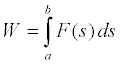Potential energy is the energy of configuration of a system. We define the change in potential energy to be the opposite of the work done by a conservative force--gravity, for example, as opposed to a non-conservative force like friction--on the components of a system during some change in the configuration of the system. As an apple falls to the ground, gravity does work on the Earth-apple system (it exerts a force through a distance), bringing their centers of mass closer, and in so doing reduces the potential energy of the Earth-apple system.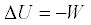and therefore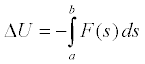which also means that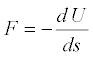Note that potential energy properly applies to a system, not to the individual components of the system. With that in mind, it is also very common to refer to the potential energy of an individual, relatively small component of a system when there exists a relatively large component. In the Earth-apple system, the force of gravity acts symmetrically on the Earth and the apple, producing a force on each of roughly one Newton. While one Newton is more than enough force to noticeably accelerate an apple, it doesn't accelerate the Earth very much at all because Earth's mass is so large. We can therefore speak of the potential energy of a small component of the system with the understanding that while such use is not completely correct, it is correct enough.

One nice thing about potential energy is that we can define the configuration of the system for which the potential energy is zero as any configuration we want. We usually pick a configuration that makes a given analysis easy. For the Earth-apple system, we would pick the configuration of the apply lying on the ground as the zero potential energy configuration. We would then say (mindful of the caveat in the paragraph above) that as the apple hangs on the tree, it has some positive amount of gravitational potential energy. As the apple falls, gravity does work on the system, the change in potential energy is negative, and the apple's potential energy is reduced to zero.

Sometimes it's not convenient to use such a configuration to define zero potential energy. In astronomical work, for example, the potential energy of a system is defined to be zero when the components are an infinite distance apart. That may seem strange at first, but it has the advantage of giving everything in the universe equal footing.

Regardless of the initial configuration used, the potential energy of a system is equal to the work done by an external force in assembling the system starting from the initial configuration.

For example, let's calculate the gravitational potential energy of the Earth-Moon system.  We claim that at infinite separation, the potential energy of the Earth-Moon system is zero.  We then find the work done to bring the Earth and Moon together.  Once we have that, we have the potential energy of the system.

If we bring the Earth and Moon together from infinite separation to distance d, we have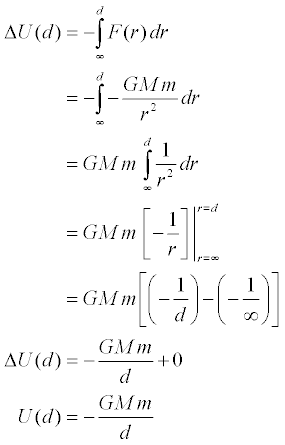The last two steps show why it is common practice to set U(∞) = 0 when great distances are involved—because the potential energy would "naturally" be zero at an infinite separation.

Now all we need are the constants:

 G = 6.6743×10-11 m3·kg-1·s-2 Universal gravitational constant2 M = 5.9736×1024 kg Mass of the Earth m = 7.3490×1022 kg Mass of the Moon d = 3.844×108 m Distance between Earth and Moon

We obtain -7.62×1028 Joules.  In other words, to move the Moon away from Earth to an infinite distance through the force of their mutual gravitational attraction, some outside agent would have to insert 7.62×1028 Joules into the Earth-Moon system.  This is equivalent to about three minutes and eighteen seconds worth of the Sun's total energy output, or roughly 150 million years worth of the energy consumed by the human population, assuming the global consumption of energy were to remain constant at its 2005 level. 3,4

Potential at a given location is the potential energy per unit mass, sort of a "specific potential energy" if you will.  Potential is defined as followswhere m0 is a unit mass. With this definition, we see that the potential of the gravitational field around a point mass is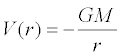Since the acceleration due to gravity is described bythen, in the same manner that force is related to potential energy, acceleration is related to potential by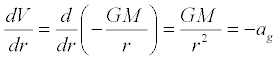and thereforeTo describe the gravitational potential of the solar system, we simply add the potentials of the Sun and the planets, taking into account the distance of each from the Sun.  We define a gravitational potential function for each astronomical body under two conditions: locations outside of the astronomical bodies and locations within those bodies. Here, we create a piecewise function such that the gravitational potential within the bodies is equal to that at their surfaces. This is not entirely meaningful, but it does make the function continuous.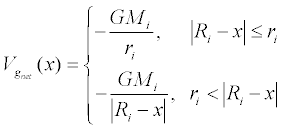where

 Vgnet(x) = Net gravitational potential, J G = 6.6743×10-11 m3·kg-1·s-2 Universal gravitational constant 2 Mi = Mass of the ith body, kg Ri = Semi-major axis of the orbit of the ith body, m ri = Radius of the ith body, m x = Radial distance from center of the Sun, m

We then sum the potentials to find the net gravitational potential.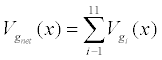where the constants for the selected bodies have the values described in the table below.4
 i Body Mass Mi (kg) Radius ri (m) Semi-major axis Ri (m) 1 Sun 1.9891×1030 6.6960×108 0 2 Mercury 3.3020×1023 2.4397×106 5.7910×1010 3 Venus 4.8685×1024 6.0518×106 1.0821×1011 4 Earth 5.9736×1024 6.3710×106 1.4960×1011 5 Moon 7.3490×1022 1.7371×106 1.4998×1011 6 Mars 6.4185×1023 3.3895×106 2.2792×1011 7 Jupiter 1.8986×1027 6.9911×107 7.7857×1011 8 Saturn 5.6846×1026 5.8232×107 1.4335×1012 9 Uranus 8.6832×1025 2.5362×107 2.8725×1012 10 Neptune 1.0243×1026 2.4622×107 4.4951×1012

Plots of the gravitational potential function over several regions of the solar system were made using Mathematica. The plots assume that all astronomical bodies lie in the plane of the ecliptic and are in conjunction on the same side of the Sun; i.e., they lie along a straight line in space with the sun located at r = 0. The values in the domain r < 0 represent the potential on the side of the Sun opposite that of the planets. The plot domains are scaled in Astronomical Units where 1 AU = 1.49598×1011 meters.5

Click on the plot thumbnail for a high resolution image best viewed with a 1280x1024 screen resolution.  The Mathematica notebook source code is available here.

Mercury through Jupiter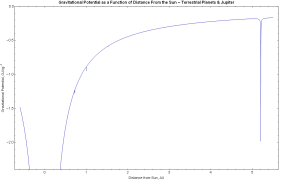The terrestrial planets